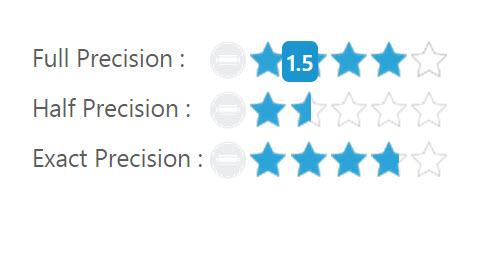# Getting Started

This section explains briefly about how to create a Rating control in your application with JavaScript. Essential JavaScript Rating helps to select the number of stars that represent Rating. Here, you can learn how to create Rating control in a real-time movie download application and also learn how to rate the application.

The following screenshot illustrates the functionality of a Rating widget with a Rating range of 0 to 5.##Create a Rating Widget

Essential JavaScript Rating widget is provided with built-in features such as precision, orientation and flexible API’s. You can create the Rating widget by using input textbox element as follows:

Create an HTML file and add the following template to the HTML file.

• html
• ``````<!doctype html>
<html>
<meta charset="utf-8" />
<title>Getting Started - RichTextEditor</title>
<script src="http://cdn.syncfusion.com/js/assets/external/jquery-1.10.2.min.js"></script>
<script src="http://cdn.syncfusion.com/18.3.0.47/js/web/ej.web.all.min.js"></script>
<body>
<! -- add rating element here -->
</body>
</html>``````

Add movies list in the table to render Rating control. Here, you can add corresponding images in the images folder and give the accurate image path.

• html
• ``````<div class="content-container-fluid">
<div class="row">
<div class="cols-sample-area">
<div style="width: 500px">
<div id="moviesTab">
<ul>
<li><a href="#steelman">Man of Steel</a></li>
<li><a href="#woldwar">World War Z</a></li>
<li><a href="#unive">Monsters University</a></li>
</ul>
<div id="steelman">
<div>
<table>
<tr>
<td class="movies-img" valign="top">
<img src="../images/rating/mos.png" alt="mos" />
</td>
<td valign="top">
<div>
Rating :
<br />
<input id="mosRating" type="text" class="rating" />
<span>Movie Info:</span>
<p>
A young boy learns that he has extraordinary powers and is not of this Earth.
</p>
</div>
</td>
</tr>
</table>
</div>
</div>
<div id="woldwar">
<table>
<tr>
<td class="movies-img" valign="top">
<img src="../images/rating/wwz.png" alt="mos" />
</td>
<td valign="top">
<div>
Rating :
<br />
<input id="wwzRating" type="text" class="rating" />
<span>Movie Info:</span>
<p>
The story revolves around United Nations employee Gerry Lane (Pitt).
</p>
</div>
</td>
</tr>
</table>
</div>
<div id="unive">
<table>
<tr>
<td class="movies-img" valign="top">
<img src="../images/rating/mu.png" alt="mos" />
</td>
<td valign="top">
<div>
Rating :
<br />
<input id="univRating" type="text" class="rating" />
<span>Movie Info:</span>
<p>
Mike Wazowski and James P. Sullivan are an inseparable pair, but that wasn't always the case.
</p>
</div>
</td>
</tr>
</table>
</div>
</div>
</div>
</div>
</div>
</div>``````

Initialize the Rating in ts file by using the `ej.Rating` method.

• javascript
• ``````/// <reference path="jquery.d.ts" />
/// <reference path="scripts/typings/ej/ej.web.all.d.ts" />

module RatingComponent {
\$(function () {
var tab = new ej.Tab(\$("#moviesTab"));
var rating = new ej.Rating(\$("#mosRating"), {
value: 2
});
var rating1 = new ej.Rating(\$("#wwzRating"), {
value: 4
});
var rating2 = new ej.Rating(\$("#univRating"), {
value: 4
});
});
}``````

Apply the following styles to show the Rating widget in the horizontal order.

• css
• ``````<style type="text/css" class="cssStyles">
.movies-img {
width: 125px;
}

font-size: 20px;
font-weight: 600;
}
</style>``````

The following screenshot displays a Rating widget.##Set the Min and Max Value

In a real-time scenario, you can extend the range by using the properties minValue and maxValue in the Rating widget.

• html
• ``````<div class="content-container-fluid">
<div class="row">
<div class="cols-sample-area">
<div class="frame">
<table>
<tr>
<td valign="top">Rate:
</td>
<td>
<input id="fullRating" type="text" class="rating" />
</td>
</tr>
</table>
</div>
</div>
</div>
</div>``````
• javascript
• ``````/// <reference path="jquery.d.ts" />
/// <reference path="scripts/typings/ej/ej.web.all.d.ts" />

module RatingComponent {
\$(function () {
var rating = new ej.Rating(\$("#mosRating"), {
value: 2,
minValue: 2,
maxValue: 10
});
});
}``````

The above code example displays the following output.##Set Precision

In a real-time movie Rating scenario, you can set precision in between two whole numbers, such as 2.5 or 3.7 and it is done using the property precision by changing the value to ej.Rating.Precision.Half or ej.Rating.Precision.Exact.

• html
• ``````<body>
<div class="content-container-fluid">
<div class="row">
<div class="cols-sample-area">
<div class="frame">
<table>
<tr>
<td valign="top">Full Precision :
</td>
<td>
<input id="fullRating" type="text" class="rating" />
</td>
</tr>
<tr>
<td valign="top">Half Precision :
</td>
<td>
<input id="halfRating" type="text" class="rating" />
</td>
</tr>
<tr>
<td valign="top">Exact Precision :
</td>
<td>
<input id="exactRating" type="text" class="rating" />
</td>
</tr>
</table>
</div>
</div>
</div>
</div>``````
• javascript
• ``````/// <reference path="jquery.d.ts" />
/// <reference path="scripts/typings/ej/ej.web.all.d.ts" />

module RatingComponent {
\$(function () {
var fullRating = new ej.Rating(\$("#fullRating"), {
value: 4,
});
var halfRating = new ej.Rating(\$("#halfRating"), {
precision: ej.Rating.Precision.Half,
value: 3.5
});
var exactRating = new ej.Rating(\$("#exactRating"), {
precision: ej.Rating.Precision.Exact,
value: 3.7
});
});
}``````

The above code example displays the following output.You can also add additional functionalities such as orientation, event tracer and API’s to the Rating.